# Concept of Dimensions

We will look at this example in order to understand the concept of dimension.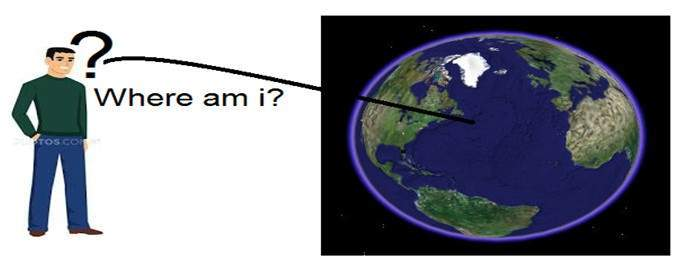Consider you have a friend who lives on moon, and he wants to send you a gift on your birthday present. He ask you about your residence on earth. The only problem is that the courier service on moon doesnot understand the alphabetical address, rather it only understand the numerical co-ordinates. So how do you send him your position on earth?

Thats where comes the concept of dimensions. Dimensions define the minimum number of points required to point a position of any particular object within a space.

So lets go back to our example again in which you have to send your position on earth to your friend on moon. You send him three pair of co-ordinates. The first one is called longitude , the second one is called latitude, and the third one is called altitude.

These three co-ordinates define your position on the earth. The first two defines your location, and the third one defines your height above the sea level.

So that means that only three co-ordinates are required to define your position on earth. That means you live in world which is 3 dimensional. And thus this not only answers the question about dimension, but also answers the reason, that why we live in a 3d world.

Since we are studying this concept in reference to the digital image processing, so we are now going to relate this concept of dimension with an image.

## Dimensions of image

So if we live in the 3d world, means a 3 dimensional world, then what are the dimensions of an image that we capture. An image is a two dimensional, thats why we also define an image as a 2 dimensional signal. An image has only height and width. An image does not have depth. Just have a look at this image below.If you would look at the above figure, it shows that it has only two axis which are the height and width axis. You cannot perceive depth from this image. Thats why we say that an image is two dimensional signal. But our eye is able to perceive three dimensional objects, but this would be more explained in the next tutorial of how the camera works, and image is perceived.

This discussion leads to some other questions that how 3 dimension systems is formed from 2 dimension.

## How does television works?

If we look the image above, we will see that it is a two dimensional image. In order to convert it into three dimension, we need one other dimension. Lets take time as the third dimension, in that case we will move this two dimensional image over the third dimension time. The same concept that happens in television, that helps us perceive the depth of different objects on a screen. Does that mean that what comes on the T.V or what we see in the television screen is 3d. Well we can yes.

The reason is that, in case of T.V we if we are playing a video. Then a video is nothing else but two dimensional pictures move over time dimension. As two dimensional objects are moving over the third dimension which is a time so we can say it is 3 dimensional.

## Different dimensions of signals

### 1 dimension signal

The common example of a 1 dimension signal is a waveform. It can be mathematically represented as

F(x) = waveform

Where x is an independent variable. Since it is a one dimension signal , so thats why there is only one variable x is used.

Pictorial representation of a one dimensional signal is given below:The above figure shows a one dimensional signal.

Now this lead to another question, which is, even though it is a one dimensional signal ,then why does it have two axis?. The answer to this question is that even though it is a one dimensional signal, but we are drawing it in a two dimensional space. Or we can say that the space in which we are representing this signal is two dimensional. Thats why it looks like a two dimensional signal.

Perhaps you can understand the concept of one dimension more better by looking at the figure below.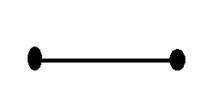Now refer back to our initial discussion on dimension, Consider the above figure a real line with positive numbers from one point to the other. Now if we have to explain the location of any point on this line, we just need only one number, which means only one dimension.

### 2 dimensions signal

The common example of a two dimensional signal is an image, which has already been discussed above.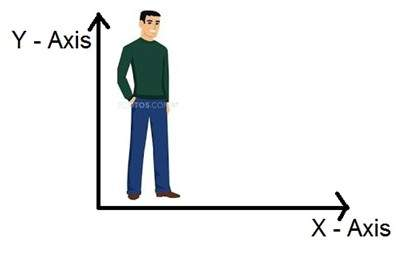As we have already seen that an image is two dimensional signal, i-e: it has two dimensions. It can be mathematically represented as:

F (x , y) = Image

Where x and y are two variables. The concept of two dimension can also be explained in terms of mathematics as: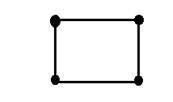Now in the above figure, label the four corners of the square as A,B,C and D respectively. If we call, one line segment in the figure AB and the other CD, then we can see that these two parallel segments join up and make a square. Each line segment corresponds to one dimension, so these two line segments correspond to 2 dimensions.

### 3 dimension signal

Three dimensional signal as it names refers to those signals which has three dimensions. The most common example has been discussed in the beginning which is of our world. We live in a three dimensional world. This example has been discussed very elaborately. Another example of a three dimensional signal is a cube or a volumetric data or the most common example would be animated or 3d cartoon character.

The mathematical representation of three dimensional signal is:

F(x,y,z) = animated character.

Another axis or dimension Z is involved in a three dimension, that gives the illusion of depth. In a Cartesian co-ordinate system it can be viewed as: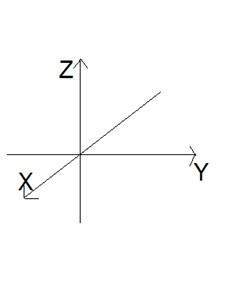### 4 dimension signal

In a four dimensional signal, four dimensions are involved. The first three are the same as of three dimensional signal which are: (X, Y, Z), and the fourth one which is added to them is T(time). Time is often referred to as temporal dimension which is a way to measure change. Mathematically a four d signal can be stated as:

F(x,y,z,t) = animated movie.

The common example of a 4 dimensional signal can be an animated 3d movie. As each character is a 3d character and then they are moved with respect to the time, due to which we saw an illusion of a three dimensional movie more like a real world.

So that means that in reality the animated movies are 4 dimensional i-e: movement of 3d characters over the fourth dimension time.